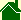#What are the problems with the Ball-of-Light Particle Model?

### New Basic Units are needed

The biggest problem with the Ball-of-Light Particle Model is it is not finished. Specifically, to finish this Grand Unification Theory, the scientific community must define new Basic Units. With the appropriate Basic Units, the equation E cross B = G is finished.

### A new proportionality constant is needed

But from a more practical view, to correlate this equation with current physics, a proportionality constant is needed. This will make it easy to convert the old physics to the new physics. There are many ways this can be accomplished. I do not wish to make the decision. It would not be appropriate for me to make.

### Model the Ball-of-Light Particle Model using a supercomputer

Finally, the equation, E cross B = G must be described in terms of a integral over a sphere. The spherical integral of E cross B will define mass. A computer model of this equation using Spherical Harmonics should be able to predict all known elementary particles.